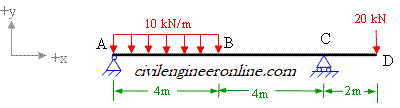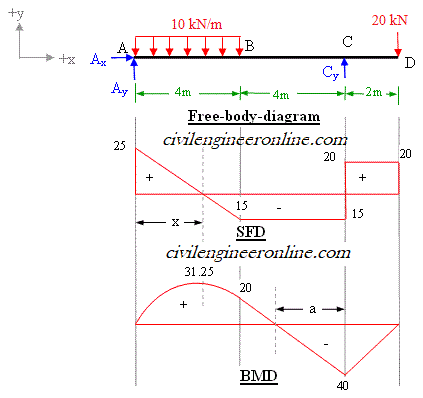Bending Moment and shear force diagram for overhanging beam

Problem 5-4

Determine the values and draw the diagrams for shear force and bending moment due to the imposed load on overhanging beam shown in figure 5-4(a) and find the position of point of contra-flexure, if any.Figure 5-4(a)

Solution:

The overhanging beam is a beam which has unsupported length on one or both sides.

Calculation of support reactions

In the case of beam shown in figure 5-4(a), there are three reaction components; Ax , Ay and Cy. The free-body-diagram is shown in figure 5-4(b).

Applying the equations of static equilibrium we get;

ΣFx = 0;  Ax = 0;                                (eq. 1)

ΣFy = 0; Ay + Cy – 10x4 – 20  = 0;

Ay + Cy = 60 kN;                 (eq. 2)

Considering z-axis passing through A, and taking moment of all the forces about z-axis (taking clockwise –ve and anticlockwise +ve);

ΣMz = 0;  Cy x 8 – 20x10 – 10x4x2 = 0;    (eq. 3)

Solving eq. 3, we get Cy = 280/8 = 35 kN;

Substituting the value of Cy in eq. 2, we get Ay = 25 kN;

Shear force calculations

In case of point load acting at a point, we should calculate shear force on both sides (left and right) of the point.

FA-left = 0;

FA-right = 25 kN;

FB = 25 – 10x4 = -15 kN;

FC-left = -15 kN;

FC-right = 25 – 40 + 35 = 20 kN;

FD-left = 20 kN;

FD-right = 20 – 20 = 0;

The values of shear force are plotted in SFD in figure 5-4(b)

Bending Moment Calculations

MA = 0;

MB = 25x4 – 10x4x2 = 20 kNm;

MC = 25x8 –10x4x6 = – 40 kNm;

The values of bending moment are plotted in BMD in figure 5-4(b)Figure 5-4(b)

Maximum +ve bending moment will occur at the point of zero shear force, which can be easily calculated by using the property of similar triangles of shear force diagram between A and B as given below;

25/x = 15/(4–x);

Which gives x = 2.5 m;

Therefore maximum +ve bending moment will occur at x = 2.5 m;

Mmax (+ve) = 25x2.5 – 10x(2.5)x(2.5/2) =  31.25 kNm

and Mmax (–ve) = – 40 kNm; at point C.

It is evident from the bending moment diagram that there is a point, at which the bending moment is equal to zero. Such a point having different nature of bending moment on its two sides (left and right) is known as point of contra-flexure.

Point of contra-flexure can be determined by writing the equation of BM for part BC and put it equal to zero;

Mx= 25x – 10*4(x–2) = 0;      x= 5.33 from A.

It can also be determined by considering the diagram between B and C and using the property of similar triangles;

40/a = 20/(4–a);

Which gives; a = 2.67m;

i.e., the point of contra-flexure is at a distance of  2.67 m from support C. This information will be helpful in providing rebars in case of reinforced concrete beams.

Excellent Calculators

Stress Transformation Calculator
Calculate Principal Stress, Maximum shear stress and the their planes

To determine Absolute Max. B.M. due to moving loads.

Bending Moment Calculator
Calculate bending moment & shear force for simply supported beam

Moment of Inertia Calculator
Calculate moment of inertia of plane sections e.g. channel, angle, tee etc.

Reinforced Concrete Calculator
Calculate the strength of Reinforced concrete beam

Moment Distribution Calculator
Solving indeterminate beams

Deflection & Slope Calculator
Calculate deflection and slope of simply supported beam for many load cases

Fixed Beam Calculator
Calculation tool for beanding moment and shear force for Fixed Beam for many load cases

BM & SF Calculator for Cantilever
Calculate SF & BM for Cantilever

Deflection & Slope Calculator for Cantilever
For many load cases of Cantilever

Overhanging beam calculator
For SF & BM of many load cases of overhanging beam

Civil Engineering Quiz
Test your knowledge on different topics of Civil Engineering

Research Papers
Research Papers, Thesis and Dissertation

List of skyscrapers of the world
Containing Tall building worldwide

Forthcoming conferences
Containing List of civil engineering conferences, seminar and workshops

Profile of Civil Engineers
Get to know about distinguished Civil Engineers

Professional Societies
Worldwide Civil Engineers Professional Societies